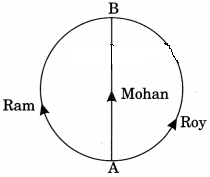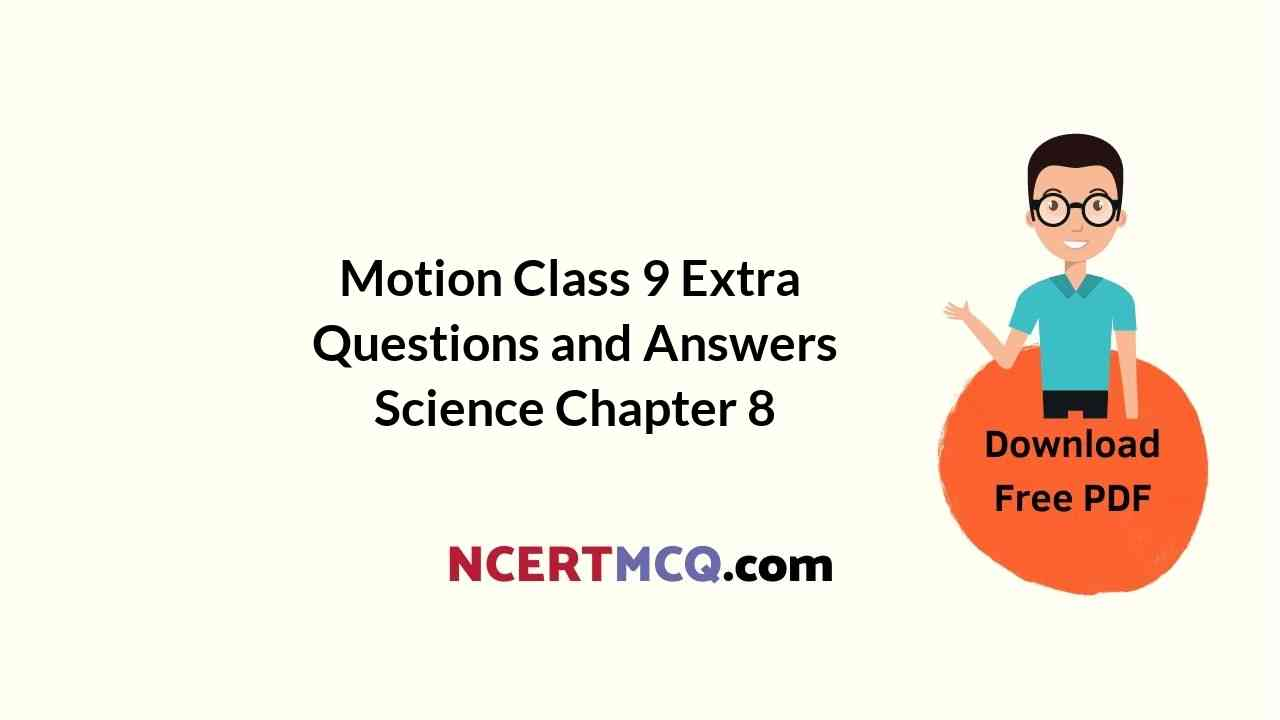In this page, we are providing Motion Class 9 Extra Questions and Answers Science Chapter 8 pdf download. NCERT Extra Questions for Class 9 Science Chapter 8 Motion with Answers will help to score more marks in your CBSE Board Exams. https://ncertmcq.com/extra-questions-for-class-9-science/

## Class 9 Science Chapter 8 Extra Questions and Answers Motion

Extra Questions for Class 9 Science Chapter 8 Motion with Answers Solutions

### Motion Class 9 Extra Questions Very Short Answer Type

Motion Class 9 Extra Questions Question 1.
Define the following terms:
(a) Distance
(b) Displacement
(c) Speed
(d) Velocity
(e) Acceleration
(f) Uniform motion
(g) Uniform circular motion
(h) Scalar quantity
(i) Vector quantity
(a) Distance: The total path length traveled by a body in a given interval of time is called distance.
(b) Displacement: The shortest distance measured from the initial to the final position of an object is known as displacement.
(c) Speed: The speed of a body is defined as the distance traveled per unit time.
(d) Velocity: Velocity is defined as displacement per unit time.
(e) Acceleration: The rate of change of velocity with respect to time.
(f) Uniform motion: A body moving in a straight line has a uniform motion if it travels the equal distance in equal intervals of tune.
(g) Non-uniform motion: A body has a non-uniform motion if it travels unequal distances in equal intervals of time.
(h) Scalar quantity: A physical quantity which is described completely by its magnitude only, is called a scalar quantity.
(i) Vector quantity: A physical quantity that has magnitude as well as direction and obeys the vector addition is called a vector quantity.

Motion Chapter Class 9 Extra Questions And Answers Question 2.
Draw position-time graph of a body that started from a position other than origin and moving with uniform speed.Class 9 Motion Extra Questions Question 3.
Give one example where the displacement is zero but the distance traveled is not zero.
When a body completes one rotation in a circular path its initial and final positions are the same and hence its displacement is zero.

Class 9 Motion Numericals Extra Questions Question 4.
The area under the velocity-time curve represents which physical quantity?
Distance and displacement.

Motion Extra Questions Class 9 Question 5.
Under what conditions, distance, and magnitude of the displacement are equal?
Distance and displacement are equal fan object move along a straight line in one direction.

Class 9 Science Chapter 8 Extra Questions And Answers Question 6.
What is the numerical ratio of average velocity to the average speed of an object when it is moving in a straight path?
In this case, both are equal, so the ratio is 1.

Motion Chapter Class 9 Extra Questions And Answers Numericals Question 7.
What does the speedometer of an automobile measure?
The speedometer of a vehicle measures its instantaneous speed.

Extra Questions Of Motion Class 9 Question 8.
Classify the following quantities on the basis of scalar quantities and vector quantities.
(a) Distance
(b) Displacement
(c) Speed
(d) Velocity
(e) Acceleration
(a) Distance: Scalar quantity
(b) Displacement: vector quantity
(c) Speed: Scalar quantity
(d) Velocity: Vector quantity
(e) Acceleration: Vector quantity

Extra Questions On Motion Class 9 Question 9.
An object starts with initial velocity y and attains a final velocity y. The velocity is changing at a uniform rate. What is the formula for calculating average speed in this situation?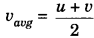Extra Questions For Class 9 Physics Motion Question 10.
What will you say about the motion of an object if its distance-time graph is a straight line with having a constant angle with a time axis?
The motion of the body is uniform motion (constant speed).

Class 9 Science Motion Extra Questions Question 11.
What will you say about the motion of a body if its velocity-time graph is a straight line parallel to the time axis?
The velocity of the body is constant.

Class 9 Science Ch 8 Extra Questions Question 12.
A physical quantity measured is -20 ms-1 It is speed or velocity?
It is velocity because velocity can be positive or negative while speed cannot be negative.

Class 9 Physics Motion Extra Questions Question 13.
A motorcycle drives from A to B with a uniform speed of 30 kmh1 and returns hack with a speed of 20 kmh1. Find its average speed.
Let distance AB = x km.
Time taken from going A to B; t1 = $$\frac {x}{30}$$ h
Time taken from goingBto A; t2 = $$\frac {x}{20}$$ h
Total time taken,t = t1 + t2 = $$\frac {x}{30}$$ + $$\frac {x}{20}$$ = $$\frac {x}{50}$$ h
total distance = x + x = 2x km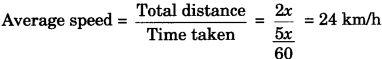∴ Average speed = 24 km/h

Ch 8 Science Class 9 Extra Questions Question 14.
A man swims in a pool of width 300 m. He covers 600 m in 10 minutes by swimming from on end to the other, and back along the same path. Find the average speed and average velocity of the man for the entire journey.
Total distance covered by the man in 10 minutes = OA + AB = 600 m
Displacement of man in 10 minutes = 0 mAverage speed = 1 m/s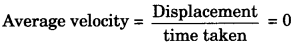Average velocity = 0 m/sExtra Questions For Class 9 Science Chapter Motion With Answers Question 15.
What is the acceleration of a body moving with uniform velocity along a straight line?
Acceleration of the body is zero as the velocity is constant.

Question 16.
Give an example of a uniform circular motion.
An athlete running on a circular track with constant speed.

Question 17.
Convert speed 54 km in meter per second.
Speed = 54 km/h =54 x $$\frac{1000 m}{3600}$$ = 15 m/s

Question 18.
What ¡s the nature of the distance-time graph for accelerated motion?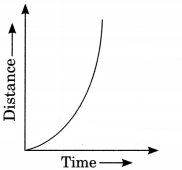### Motion Class 9 Extra Questions Short Answer Type 1

Question 1.
Distinguish between speed and velocity.
Speed:

• Speed is the ratio of distance and time.
• Speed is always positive
• Speed is a scalar quantity.

Velocity:

• Velocity is the ratio of displacement and time.
• Velocity may be negative or positive.
• Velocity is a vector quantity.

Question 2.
Distinguish between distance and displacement.
Distance

• It is the actual length of the path covered by a moving body.
• It is always positive or zero.
• It is a scalar quantity.

Displacement:

• It is the shortest distance measured between the initial and final positions.
• It may be positive, negative, or zero.
• it is a vector quantity.

Question 3.
Write down the SI unit of the following quantities:
(a) Displacement
(b) Speed
(c) Velocity
(d) Acceleration
(a) m
(b) m/s
(c) m/s
(d) m/s2

Question 4.
Distinguish between uniform motion and non-uniform motion.
1. Uniform motion:
A body moving in a straight line has a uniform motion if it travels the equal distance in equal intervals of time

2. Non.uniform motion:
A body has a non-uniform motion if it travels the unequal distance in equal intervals of time

Question 5.
Distinguish speed at any instant and average speed.
1. Instantaneous speed:
The speed at any particular instant is known as instantaneous speed.

2. Average speed:
Average speed is the ratio of total distance traveled by a body and time taken to travel that distance.

Question 6.
Draw a velocity versus time graph of a stone thrown vertically upwards and then coming downwards after attaining the maximum height.
velocity-time graph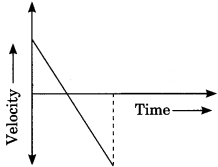Question 7.
What is uniform circular motion? How is uniform circular motion regarded as an acceleration motion? Explain.
When an object is moving in a circular path with a constant speed, the motion of an object is said to be uniform circular motion. When a body has a uniform circular motion, its velocity changes due to the continuous change in the direction of its motion. Hence, the motion of the body is accelerated motion.

Motion Class 9 Extra Questions Numericals

Question 8.
A person travels a distance of 4.0 m towards the east, then turns left and travels 3.0 m towards the north.
1. What is the total distance traveled by the person? North
2. What is his displacement?
1. Total distance = OA + AB
= 4m + 3m
Total distance = 7m2. Total displacement = OB = $$\sqrt{(O A)^{2}+(A B)^{2}}$$
=$$\sqrt{(4)^{2}+(3)^{2}}$$ = $$\sqrt{25}$$ = 5
Displacement = 5 m

Question 9.
A person travels on a semi-circular track of radius 50 m during a morning walk. If he starts from one end of the track and reaches the other end, calculates the distance traveled and displacement of the person.
Let the person start moving from A and reach B via O.
The distance travelled by the person
= Length of track = πr
= $$\frac{22}{7}$$ x 50 m = 157.14m
Distance = 157.14 m
The displacement is equal to the diameter of the semi-circular track joining A to B via O.
= 2r = 2 x 50 m = 100m
∴ Displacement = 100 m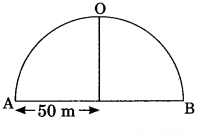Question 10.
Dinesh takes 20 minutes to cover a distance of 5 km on a bicycle. Calculate his average speed in
1. m/s
2. km/h
1. Average speed in metre/second
Distance travelled by Dmesh = 5 km = 5 x 1000 m = 5000 m
time taken (t) = 20 min = 20 x 60 seconds = 1200 seconds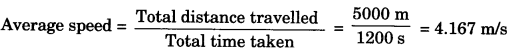2. Average speed in kilometre/hour
Average speed = $$\frac{5 \mathrm{km}}{\frac{20}{60} \mathrm{h}}$$ = 15 km/h
∴  Average speed = 15 km/h

Question 11.
A train starting from a railway station and moving with uniform acceleration attains a speed of 20 m/s in 10 seconds. Find its acceleration.
Given,
initial velocity, u = 0 m/s
Final velocity, υ = 20 m/s
time taken, t = 10 s
Acceleration, a = $$\frac{v-u}{t}=\frac{20-0}{10}$$
∴ a = 2 m/s2

Question 12.
A car moving with a speed of 72 km/h is brought to rest in 10 seconds by applying brakes. Find the magnitude of average retardation due to brakes and distance traveled by car after applying the brakes.
Given, initial velocity = 72 km/h = 72 x $$\frac{5}{18}$$ = 20 m/s
Final velocity = 0 m/s
Time taken = 10s.

1. Retardation
Acceleration, a = $$\frac{υ – u}{t}$$
a = $$\frac{0 – 20}{10}$$ = -2m/s2
∴ Retardation = 2 m/s2

2. Distance travelled using equation, υ2 – u2 = 2as
(0)2 – (20)2 = 2 (-2)s
∴ s = 100 m

Question 13.
The velocity of a particle moving along a straight line in a certain time interval is shown below. What is the distance traveled during acceleration?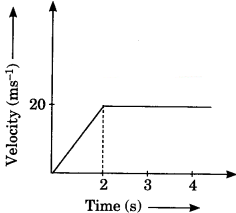Here particle accelerates for 2 seconds.
Distance traveled during acceleration = area under υ – t graph for 2 sec.
$$\frac{1}{2}$$ x Base x Height = $$\frac{1}{2}$$ x 2 x 20 = 20m

Question 14.
A girl walks along a straight path to drop a letter in the letterbox and comes back to her initial position. Her displacement—
The time graph is shown in the figure. Plot a velocity-time graph for the same.
Velocity-time graphQuestion 15.
Obtain a relation for the distance traveled by an object moving with uniform acceleration in the time interval between the 4th and 5th seconds.
Using equation, s = ut + $$\frac{1}{2}$$ at2
Distance travelled in 5 s; s1 = u x 5 + $$\frac{1}{2}$$ a(5)2
s1 = 5u + $$\frac{25a}{2}$$

Distance travelled in 4 s; s2 = u x 4 + $$\frac{1}{2}$$ a(4)2
s2 = 4u x 8a
Distance travelled in the interval between 4th and 5th sec.
= s1 – s1 = (5u + $$\frac{25a}{2}$$) – (4u + 8a)
∴ Distance travelled = u + $$\frac{9a}{2}$$

### Motion Class 9 Extra Questions Short Answer Type 2

Question 1.
Draw a position-time graph of for
(a) rest
(b) uniform motion
(c) Non-uniform motion
(a) Position – time graph for rest(b) Position – time graph for uniform motion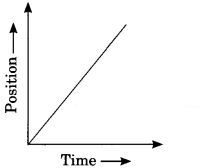(c) Position – time graph for non-uniform motionQuestion 2.
A draw velocity-time graph for
(a) Uniform motion
(b) Uniform acceleration
(c) Uniform retardation
(a) Velocity – time graph for uniform motion (Const. speed)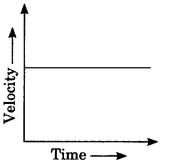(b) Velocity – time graph for uniform acceleration (Const. acceleration)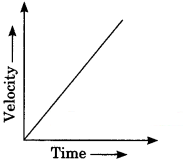(c) Velocity – time graph for uniform retardation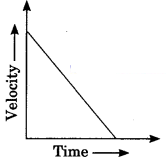Question 3.
Explain the difference between the two graphs.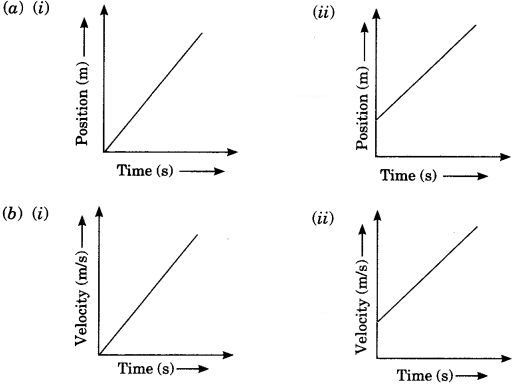(a) In the first graph, the body is starting its motion from origin but in the second graph, the body is starting its motion from any other point.
(b) In the first graph, the body has zero initial velocity but in the second graph the body has non-zero initial velocity.

Question 4.
Two stones are thrown vertically upwards simultaneously with their initial velocities u1 and u2 respectively. Prove that the heights reached by them would be in the ratio of $$\frac{1}{2}$$
(Assume upward acceleration is – g and downward acceleration to be +g).
The maximum height reached by the stone can be calculated by the formula
υ2 – u2 = 2as
Here, υ = 0 and a= – g
Applying this equation for the first ball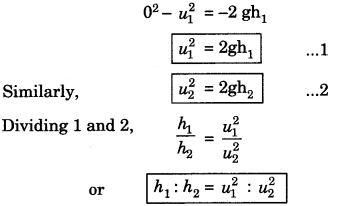Motion Class 9 Extra Questions Numericals

Question 5.
Study the velocity-time graph of a traveling metro train as shown in the figure. What is the acceleration of the train
(a) during the first two seconds?
(b) between the second and tenth seconds?
(c) during the last two seconds?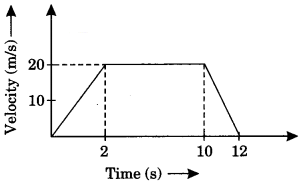(a) Acceleration, a = $$\frac {v – u}{t}$$
Here, u = 0, u = 20m/s and t = 2s
a = $$\frac {20 – 0}{2}$$ = 10 m/s2

(b) Here, u = 20m/s, υ = 20m/s and t = 8s
a = $$\frac {20 – 0}{2}$$ = 0 m/s2

(c) Here, u = 20 m/s, u = 0 m/s and t = 2s
a = 0 – 20 = – 10 m/s2

Question 6.
An electron moving with a velocity of 5 x 10 ms1 enters into a uniform electric field and acquires a uniform acceleration of 10 ms2 in the direction of its initial motion.
1. Calculate the time in which the electron would acquire a velocity double its initial velocity.
2. How much distance would the electron cover in this time?
Given, u =5 x 10 m/s2
a = 104 m/s2

1. From υ = u + at
take υ = 2u
2u = u + at
u = at
5 x 104 = 104 x t
∴ t = 5s

2. From s = ut + $$\frac{1}{2}$$ at2
s = 5 x 104 x 5 + $$\frac{1}{2}$$ x 104 x (5)2
∴ s = 37.5 x 104 m

Question 7.
The motion of a train is described by the velocity-time graph as shown in the figure given below.Find distance travelled by car between
1. 0 to 20 s
2. 20 s to 50 s
Distance travelled = Area of velocity – time curve
1. For (0 to 20 s)
Distance = Area of ΔOAC = $$\frac{1}{2}$$ x OC x AC = $$\frac{1}{2}$$ x 20 x 10 = 100 m

2. For (20 s to 50 s)
Distance = Area of rectangle ABDC + Area of ΔBED
Distance = AC x CD + $$\frac{1}{2}$$ DE x DB = 10 x (40 – 20) + $$\frac{1}{2}$$ x 10 x 5 = 225m

### Motion Class 9 Extra Questions Long Answer Type

Question 1.
Derive an expression for three equations of motion for uniform accelerated motion graphically.
Equation of motion by graphical method
Let us consider a body is moving with acceleration where u is initial velocity and u is final velocity, s is the displacement of object and t is a time interval.1. υ = u + at
We know that slope of υ – t graph gives acceleration so slope
= a = $$\frac{v-u}{t-0}$$
a = $$\frac{v-u}{t}$$
∴ υ = u + at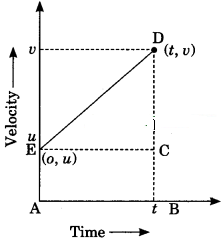2. s = ut + $$\frac{1}{2}$$ at2
We know that area under u – t graph gives the displacement.
Area = s = area of triangle CDE + area of rectangle ABCE
s = ut + $$\frac{1}{2}$$ x t x (υ – u) from (υ – u = at)
Putting the value of υ – u
s = ut + $$\frac{1}{2}$$ at2

3. υ2 – u2 = 2as
We know that area under υ – t graph gives displacement
Area = s = area of trapezium ABDE
s = $$\frac{1}{2}$$ x (υ+u) x t From I (t = $$\frac{υ – u}{a}$$)
Putting the value of t.
υ2 – u2 = 2as

### Motion Class 9 Extra Questions HOTS

Question 1.
The distance-time graph of two trains is given below. The trains start simultaneously in the
same direction.
1. How much ahead of A is B when the motion starts?
2. What is the speed of B ?
3. When and where will A catch B?
4. What is the difference between speeds of A and B?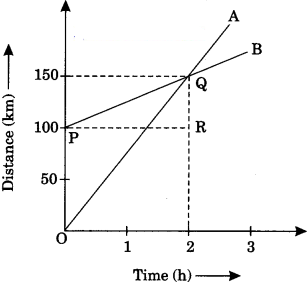5. Is the speed of both the trains uniform or non-uniform? Justify your answer.

1. 100km
2. Speed of B, υ = $$\frac{x_{2}-x_{1}}{t}$$ = $$\frac{150-100}{2-0}$$ = 25 km/h
3. After 2 hours, A catches B at 2. i.e.,150 km from origin 0.
4. The slope of the distance-time graph of A is greater than that of B. Therefore, the speed of A is greater than
speed B. Speed of A = Slope of OQ = $$\frac{150}{2}$$ = 75 km/h
Difference between speed of A and B =75 km/h – 25 km/h = 50 km/h
5. The speed of both trains is uniform.

Question 2.
A car starts from rest and moves along the x-axis with constant acceleration 5 ms2 for 8 seconds. If it then continues with constant velocity, what distance will the car cover in 12 seconds since it started from rest?
Given,
u = 0, a = 5ms-2, t1 = 8s and t2 = 12s.
Total distance = distance travelled during acceleration + distance travelled during constant velocity
s = s1 + s2

For S1
s1 = u1t1 + $$\frac{1}{2}$$ $$a t_{1}^{2}$$
s1 = $$\frac{1}{2}$$ x 5 x (8)2
s1 = 160 m

For S1
Applying υ = u + at
υ = 0 + (5 x 8)
u = 40 m/s
s1 = ut2
s2 = 40 x 12
s2 = 480 m
Total distance, s = s1 + s2
s = 160 + 480 = 640m

Question 3.
An object is dropped from rest at a height of 150 m and simultaneously another object is dropped from rest at a height 100 m. What is the difference in their heights after 2s if both the objects drop with the same acceleration? How does the difference in heights vary with time?
At t = 0, difference in heights of two stones A and B
= 150 – 100 = 50 m
In t = 2s, distance through which each stone falls is –
s = ut + $$\frac{1}{2}$$ at2 = 0 x $$\frac{1}{2}$$ x 10 x (2)2 = 20m
∴ Difference in heights of two stones A and B
= (150 – 20) – (100 – 20) = 50
The difference in heights will remain the same at all times during their fall.

Question 4.
An object starting from rest travels 20 m in the first 2s and 160 m in the next 4s. What will be the velocity after 7s from the start?
Given, the object travels 20 m first 2 s and 160 m in the next 4s.
From s = ut + $$\frac{1}{2}$$ at2
In first 2 s, s = 20 m
20 = 0 + $$\frac{1}{2}$$ a(2)2
20 = 2 a
∴ a = 10 m/s2
Velocity at the end of 2s is υ = u + at
υ = 0 + 10 x 2 = 20 m/s

In next 4 s,
s = ut + $$\frac{1}{2}$$ a’t2
160 = 20 x 4 + $$\frac{1}{2}$$ a’(4)2
80 = 8 a’
a’ = 10 m/s2

### Motion Class 9 Extra Questions Value Based (VBQs)

Question 1.
Sumeet and Jaikishan are close friends. Sumeet is a science graduate and Jaikishan is a commerce graduate. Sumeet finds that while driving on a clear highway, Jaikishan often exceeds the speed limit and argues that there is no harm in doing so when the road is clear. Sumeet does not agree with him and tells him that with an increase in speed, the stopping distance of the car would increase and he would not be able to manage if someone appears suddenly on the way.

1. is Sumeet right in his statement?
2. What values are displayed by Sumeet in his statement?
3. How is stopping distance related to the speed of the vehicle?

1. Yes, Sumeet is absolutely right.
2. Sumeet is an intelligent boy and he can apply his knowledge of science in daily life.
3. Stopping distance (velocity)2

Question 2.
Raju and Raman are close friends. One day they went for a picnic. Raju was driving the car. When Raman saw the speedometer, he found that the speed of the car was more than the allowed speed limit. Raman advised Raju to reduce speed to avoid the punishment of Overspeed.

1. What is the function of the speedometer?
2. What values are displayed by Raman?

1. Speedometer provides instantaneous speed. B
2. Raman is a careful and law-abiding person.

Question 3.
Three friends Ram, Mohan, and Roy have to reach point B from point A. The path taken by them is shown in the figure.

1. Distinguish between distance and displacement.
2. If all of them travel at the same speed, who will reach first at point B and why?